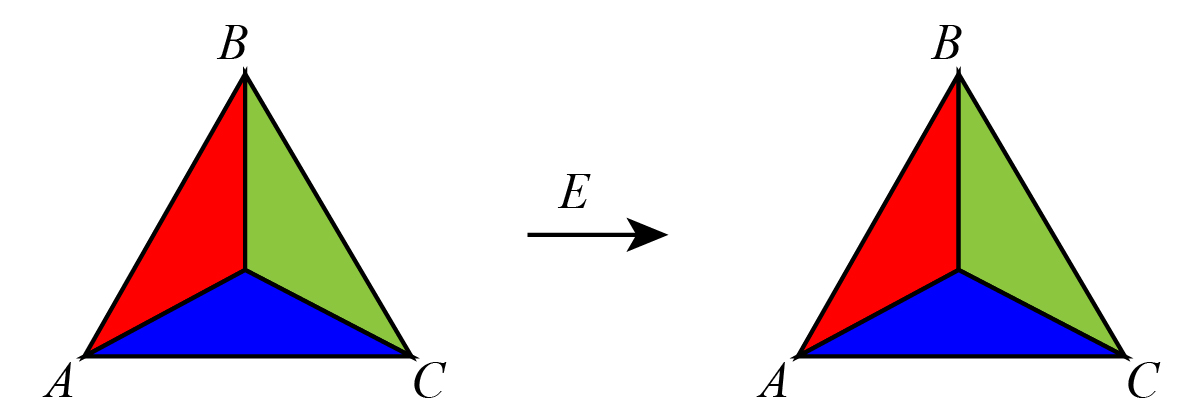### Solution Found!# With pictures and words, describe each symmetry in D3 (the

Chapter 1, Problem 1E

(choose chapter or problem)

Get Unlimited Answers! Check out our subscriptions
QUESTION:

With pictures and words, describe each symmetry in $$D_3$$ (the set of symmetries of an equilateral triangle).

QUESTION:

With pictures and words, describe each symmetry in $$D_3$$ (the set of symmetries of an equilateral triangle).

Step 1 of 3
Here the objective is to list out all the six elements in $$D_3$$.
Consider the first element E is the rotation by an angle of $$0^\circ$$ that is no change in position as shown in Figure below.